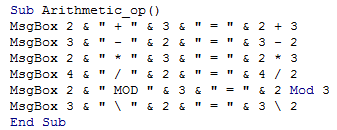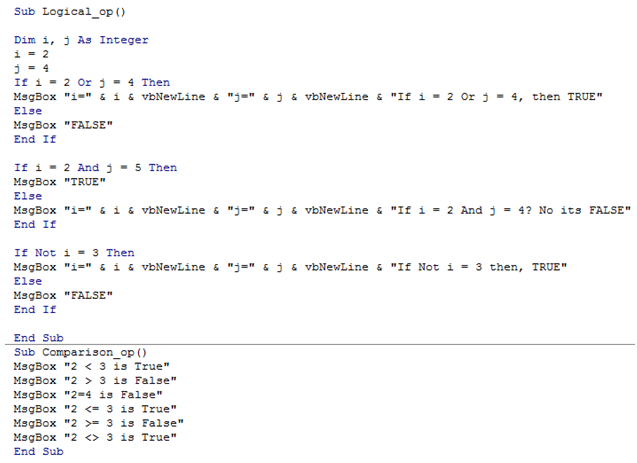## Vba operators in Excel

Operators are imporatant in any calculation and Excel is no exception. They are important in writing any VBA program.

There are mainly three types of operators used in Excel VBA appplication which are discussed as follows.

Arithmetic Operators

Arithmetic Operators ae used to execute mathematical calculation in the program

The important arithmetic operators are as follows

"+" Plus: Addition of two or more numbers (int,long,double)

"-" Minus:� Subtraction of two or more numbers (int,long,double)

"*" Product:� Product of two numbers

"/" Division:�Returns quotient of two numbers:�

MOD (Modulus):�Returns Remainder from division of two numbers�

"\" Integer Division:�Returns Integer quotient discarding decimal part

Logical Operators

Logical Operators are used to perform logical operations on both text and numbers.

Logical Operators are generally used with "IF" statement to check two or more conditions logically.

OR: Returns TRUE if either of the conditions are true.

AND: Returns TRUE if both the conditions are true.

NOT: Compliment of the conditional expression.

Comparison Operators

Comparison Operators are used to compare two variables.

Most of the operations are related to numerical variables."=" is the only operator that is used with String variables.

<: Less than

<=: Less than or equal to

>: Greater than

>=: Greater than or equal to

"=": Equal to

<> : Not Equal to

The following Code illustrates this

1. Sub Arithmetic_op()
2. MsgBox 2 & " + " & 3 & " = " & 2 + 3
3. MsgBox 3 & " - " & 2 & " = " & 3 - 2
4. MsgBox 2 & " * " & 3 & " = " & 2 * 3
5. MsgBox 4 & " / " & 2 & " = " & 4 / 2
6. MsgBox 2 & " MOD " & 3 & " = " & 2 Mod 3
7. MsgBox 3 & " \ " & 2 & " = " & 3 \ 2
8. End Sub

Logical Operation

1. Sub Logical_op()
2. Dim i, j As Integer
3. i = 2
4. j = 4
5. If i = 2 Or j = 4 Then
6. MsgBox "i=" & i & vbNewLine & "j=" & j & vbNewLine & "If i = 2 Or j = 4, then TRUE"
7. Else
8. MsgBox "FALSE"
9. End If
10. If i = 2 And j = 5 Then
11. MsgBox "TRUE"
12. Else
13. MsgBox "i=" & i & vbNewLine & "j=" & j & vbNewLine & "If i = 2 And j = 4? No its FALSE"
14. End If
15. If Not i = 3 Then
16. MsgBox "i=" & i & vbNewLine & "j=" & j & vbNewLine & "If Not i = 3 then, TRUE"
17. Else
18. MsgBox "FALSE"
19. End If
20. End Sub

Comparison Operation

1. Sub Comparison_op()
2. MsgBox "2 < 3 is True"
3. MsgBox "2 > 3 is False"
4. MsgBox "2=4 is False"
5. MsgBox "2 <= 3 is True"
6. MsgBox "2 >= 3 is False"
7. MsgBox "2 <> 3 is True"
8. End Sub

A preview of the Editor is shown below